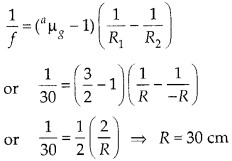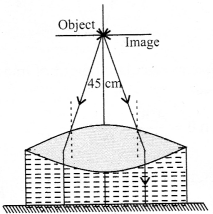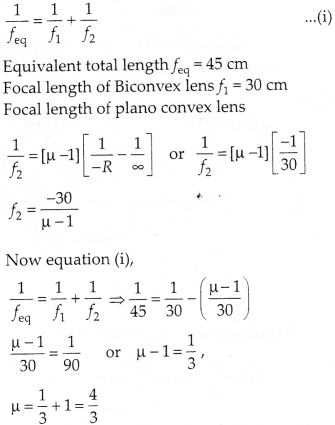Enlightened

# Question 38: NCERT Solutions for 12th Class Physics: Chapter 9-Ray Optics and Optical Instruments

• 0

Question 38: NCERT Solutions for 12th Class Physics: Chapter 9-Ray Optics and Optical Instruments

Figure shows an equiconvex lens (of refractive index 1.50) in contact with a liquid layer on top of a plane mirror. A small needle with its tip on the principal axis is moved along the axis until its inverted image is found at the position of the needle. The distance of the needle from the lens is measured to be 45.0 cm. The liquid is removed and the experiment is repeated. The new distance is measured to be 30.0 cm. What is the refractive index of the liquid?Share

1. Solution:
Let us first consider the situation when there is no liquid between lens and plane mirror and the image is formed at 30 cm i.e., at the position of object. As the image is formed on the object position itself, the object must be placed at focus of Biconvex lens.ƒ0 = 30 cm Radius of curvature of convex lens can be calculatedNow a liquid is filled between lens and plane mirror and the image is formed at position of object at 45 cm. The image is formed on the position of object itself, the object must be placed at focus of equivalent lens of Biconvex of glass and Plano convex lens of liquidCheck the complete chapter with solutions.

NCERT Solutions for 12th Class Physics: Chapter 9-Ray Optics and Optical Instruments

• 0